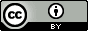## Wednesday, June 18, 2014

### Peer instruction: writing good (chemistry) questions

A good peer instruction question is a question that facilitates a good discussion and is just difficult enough that about half the students get it wrong on the first vote. Such questions are hard to write. Be prepared to spend time and mental effort on this, and to revise or replace questions in coming years as you get feedback from the students.

If you ask bad questions students will grow bored quickly. Avoid simple recall questions (the particles orbiting the atomic nucleus are called ...?), questions that can be answered by a simple Google query, or questions that require a calculator (how many moles in 32 g of Bi?), none of which facilitates discussion.

Below I collected some example of questions I have used in my thermodynamics class:

I always include a "don't know" option and tell them: "if you really have no idea how to attack the problem, don't guess; let me know by voting "don't know".

Also, don't over-define the problem. It's OK to leave things out (such as underlying assumptions or simplifications) and let them ask you as needed.

Slide 1. This is a typical multiple choice question where the students are presented with several answers that are quite different from one another. These are hard to write because it is difficult to come up with several different but plausible answers (see also slide 6).

I use this question to address two common mistakes I have seen my students make: A looks good because they confuse the entropy of the universe (which goes to a maximum according to the second law) with the entropy of the system. C looks good because students confuse the standard free energy change with the free energy of the system.  I also use graphs and pictures whenever possible.

Slide 2. A better approach is often to formulate questions such that the possible answers are "more", "less" or "the same" (or "increase", "decrease", "stay unchanged").  This is probably the most used peer instruction question format.  The challenge then is to formulate the question such that one of the wrong answers looks most plausible.  Here the two complexes look very similar so option C is somewhat attractive.

Slide 3. Another variant of more/less/the same

Slide 4. Another approach is to ask which X has the largest/smallest value of Y. Here it is important that the choices are sufficiently different so that the answer can be obtained without looking anything up or memorizing it. In this case each molecule has a different number of polar atoms. The question can also be rewritten as a ranking problem, such as that shown in the next slide.

Slide 5. Ranking in order of increasing/decreasing X. I use molecular models rather than chemical formulas so that they have to deduce the molecular charge themselves and get a sense of the relative size of the molecules; both if which are key to answering the question correctly.

Slide 6. This is an example of how to cover equations without asking questions that require a calculator.  Also, since it harder to formulate plausible wrong questions I ask for the answer that is not correct.

Slide 7. This question appears to break the "calculator rule".  However, I have taught them how to estimate the result to within an order of magnitude and formulated the question accordingly.  I use this approach only for the 1-2 most important equations covered in the course and I ask about it frequently - it is a difficult skill to learn.

Experiments and Simulations
The above type of questions can be greatly improved by including (movies of) experiments or simulations. It makes the topic less abstract and more relevant and it addresses exactly what chemistry is all about: explaining observations in terms of the behavior of atoms and molecules.

I typically search Youtube for "xxx experiment" or "xxx simulation" and then capture the part of the video I want with screencasting software such as Screenflow or Camtasia and insert the resulting movie in a Powerpoint slide. The free program Molecular Workbench also has en extensive library of simulations and allows you to make your own.  Of course you can perform the experiment in class or record your own experiments in lab.

Here is a video of some of the questions I have made for my thermodynamics course